Next: TT800 and Mersenne Twister Up: Random Number Generators Previous: Inversive Congruential Generators

## Tausworthe Generators

Tausworthe Generator (TG)  is a kind of multiplicative recursive generator (see Section 3.1) which produces random bits. It has the following form: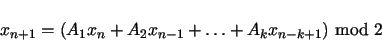where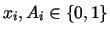for all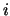.

The theory behind TG is related to irreducible primitive polynomials over GF(2). A polynomial over Galois field of order 2 (GF(2)) is a polynomial whose coefficients are either 0 and 1. Such a polynomial is irreducible primitive if it does not have nontrivial factors like 1 and has order of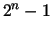for some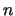. The order of a polynomial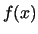over GF(2) is the smallest integer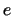for whichdivides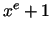. For example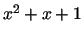is irreducible primitive with order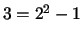, while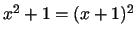is not even irreducible.

When the characteristic polynomial of the above form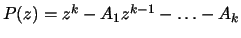is an irreducible primitive and the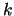initial seeds are not all zero, the corresponding TG will have a period of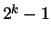. To obtain random numbers instead of bits, we can output the decimal values of every-consecutive bits.

Since TG only produces bits, it is too slow to be useful. A technique to speed up is to use a special form called trinomial-based TG. A trinomial is a polynomial with only three coefficients being nonzero, so a trinomial-based TG has only two of the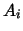coefficients of the characteristic polynomial being nonzero. This results in a very fast, hardware-based implementation technique called Feedback Shift Register (FSR) which involves shift, AND, and XOR operations on a small set of-bit registers. However, TGs based on this special form are known to have statistical defects, so in practice the outputs of two or more trinomial-based TGs are combined using bitwise XOR operations to provide the final output. As with LCGs, arbitrarily chosen parameters will yield poor TGs, and not all combinations of any three good TGs necessarily create a better TG, therefore, in this project we implemented a 3-combined TG based on parameters from  with period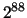The main attraction of TGs lies in its independence of word size of target hardware and some of the variants have strong cryptographic safety (see Section 3.5)Next: TT800 and Mersenne Twister Up: Random Number Generators Previous: Inversive Congruential Generators
2001-05-30